### Home > CALC > Chapter Ch2 > Lesson 2.2.3 > Problem2-81

2-81.
1. A helium balloon is released from the ground and floats upward. The height of the balloon is shown at the following times: Homework Help ✎

 Time (sec) Height (feet) 0 1 2 3 4 5 6 7 8 9 10 0 50 98 144 188 230 270 308 344 378 410

1. What was the average velocity over the first 10 seconds of the balloon's flight? Over the first 5 seconds?

2. Find the finite differences for the heights. How is the velocity changing? Explore this using the 2-81 eTool (Desmos).

3. What do the finite differences tell you about the height function for the balloon?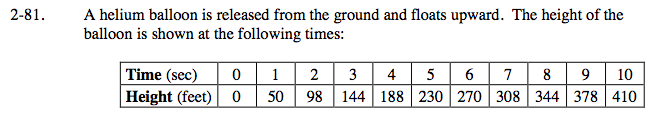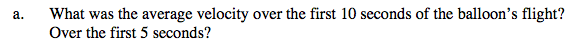In the case of the helium balloon.

$\text{average velocity }=\frac{\Delta\text{height}}{\Delta\text{time}}$

average velocity between t = 0 and t = 10:

$\frac{h(10)-h(0)}{10-0}=\frac{410-0}{10-0}=41 \text{ft/sec}$

Now find the average velocity between t = 0 and t = 5.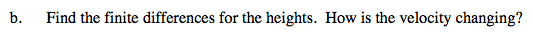This is an example of a finite difference table.

Use the eTool below to explore this.
Click on the link below to view the full version of the eTool. Calc 2-81 HW eTool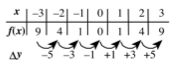In this story, finite differences (Δy) represent the average velocity over consecutive one second intervals.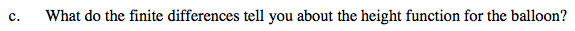Is the height of the balloon increasing, decreasing or both? How do finite differences help you answer this?